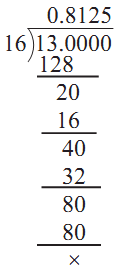# Decimal Form of Rational Number

The process of expressing a rational number into decimal form is to carry out the process of long division using decimal notation.

For example, 13/16 = 0.8125In cases where the denominator has factors other than 2 or 5, the decimal representation starts repeating. Such decimals are called non-terminating repeating decimals.

The decimal representation of a rational number is

1. either a terminating decimal (and the remainder is zero after a finite number of steps)
2. or a non-terminating repeating decimal (where the division will never end)

Thus, a rational number is either a terminating decimal or a non-terminating repeating decimal.